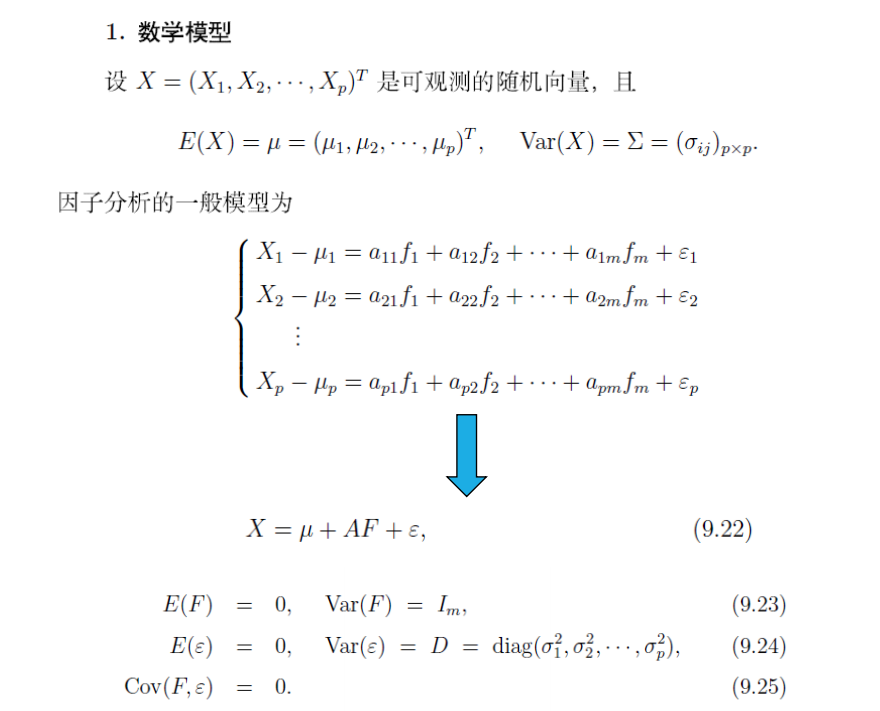• ## 因子分析法

千次阅读 2021-04-02 22:57:56
因子分析法 今天博主想介绍一个很经典的降维方法因子分析法，很多人可能降维第一想到的是主成分分析法，确实主成分分析法是很经典的， 因子分析是指研究从变量群中提取共性因子的统计技术。最早由英国心理学家C.E....
因子分析法
今天博主想介绍一个很经典的降维方法因子分析法，很多人可能降维第一想到的是主成分分析法，确实主成分分析法是很经典的，
因子分析是指研究从变量群中提取共性因子的统计技术。最早由英国心理学家C.E.斯皮尔曼提出。他发现学生的各科成绩之间存在着一定的相关性，一科成绩好的学生，往往其他各科成绩也比较好，从而推想是否存在某些潜在的共性因子，或称某些一般智力条件影响着学生的学习成绩。因子分析可在许多变量中找出隐藏的具有代表性的因子。将相同本质的变量归入一个因子，可减少变量的数目，还可检验变量间关系的假设。
简而言之，因子分析法，认为很多变量之间具有相关性，而这种相关性本质上是指多个变量可能存在着一个共同的影响因子，这一个影响因子就可以通过多个函数得到多个变量的近似。那么我们就可以用这一个隐变量去表示多个变量的信息。
因子分析 – 降维的一种方法，是主成分分析的推广和发展 – 是用于分析隐藏在表面现象背后的因子作用的统计模型。试图用最少个数的不可测的公共因子的线性函数与特殊因子之和来描述原来观测的每一分量 主要用途 – 减少分析变量个数 – 通过对变量间相关关系的探测，将原始变量分组，即将相关性高的变量分为一组，用共性因子来代替该变量 – 使问题背后的业务因素的意义更加清晰呈现
数学模型表示：如果你听懂了上面原理的介绍，那么对于上面的数学模型会有一个很好的理解，所以，很多时候，我认为学习要真懂。如上述。F就是我们最终得到的因变量，而对于A则是各个变量对于因变量的一个权重，对于隐变量和变量的关系十分重要。
因子分析法基本原理在对某一个问题进行论证分析时，采集大量多变量的数据能为我们的研究分析提供更为丰富的信息和增加分析的精确 度。然而，这种方法不仅需要巨大的工作量，并且可能会因为变量之间存在相关性而增加了我们研究问题的复杂性。因子分析法就是从研究变 量内部相关的依赖关系出发，把一些具有错综复杂关系的变量归结为少数几个综合因子的一种多变量统计分析方法。这样我们就可以对原始的 数据进行分类归并，将相关比较密切的变量分别归类，归出多个综合指标，这些综合指标互不相关，即它们所综合的信息互相不重叠。这些综 合指标就称为因子或公共因子。因子分析法的基本思想是将观测变量进行分类，将相关性较高，即联系比较紧密的分在。 同一类中，而不同类变量之间的相关性则较低，那么每一类变量实际上就代表了一个基本结构，即公共因子。
对于所研究的问题就是试图用 最少个数的不可测的所谓公共因子的线性函数与特殊因子之和来描述原来观测的每一分量。这样，就能相对容易地以较少的几个因子反映原资 料的大部分信息，从而达到浓缩数据，以小见大，抓住问题本质和核心的目的。因子分析法的核心是对若干综合指标进行因子分析并提取公共 因子，再以每个因子的方差贡献率作为权数与该因子的得分乘数之和构造得分函数。因子分析法的数学表示为矩阵:，即:(kp)(1式)模型中，向 量X是可观测随机向量，即原始观测变量。F是X的公共因子，即各个原观测变量的表达式中共同出现的因。
展开全文机器学习 数学建模
• SPSS中因子分析法的操作步骤，深入浅出分析了SPSS中因子分析法的基本原理，让初学者掌握和理解SPSS中因子分析。
•文档
• MATLAB实现平行因子分析法（PARAFAC）
• 介绍主成分分析法与因子分析法的关系，利用spss软件，介绍一个案例主成分
• 因子分析法之因子旋转 1.因子旋转及其意义 建立因子分析模型的目的不仅是要找出公因子以及对变量进行分组，更重要的是要知道每个公因子的意义，以便对实际问题做出科学分析。因子旋转即对因子载荷矩阵A，用一个正交...
因子分析法之因子旋转
1.因子旋转及其意义
建立因子分析模型的目的不仅是要找出公因子以及对变量进行分组，更重要的是要知道每个公因子的意义，以便对实际问题做出科学分析。因子旋转即对因子载荷矩阵A，用一个正交矩阵T右乘A实现对因子载荷矩阵的旋转（一次正交变换即对应坐标系的一次旋转），旋转后因子载荷矩阵结构简化，更容易对公因子进行解释。
结构简化就是重新分配每个因子所解释方差的比例，使每个变量仅在一个公因子上有较大的载荷，在其他公因子上的载荷较小，即是使因子载荷矩阵每行或者每列元素的平方值向0与1两极分化。
2.几个重要概念
2.1.因子载荷
因子载荷是连接变量与公因子的纽带，例如标准化处理的变量X=（x1,x2…xp）,因子分析的一般模型为： x1=a11f1+a12f2+…+a1mfm+

ϵ

\epsilon

1x2=a21f1+a22f2+…+a2mfm+

ϵ

\epsilon

2…xp=ap1f1+ap2f2+…+apmfm+

ϵ

\epsilon

p aij即因子载荷，f1,f2…fm是公因子，

ϵ

\epsilon

i称为特殊因子，实际分析时候可以忽略不计。
2.2.变量共同度
变量共同度也称为公因子方差，表达式为：

h

i

2

h^2_ i

＝

∑

j

=

1

m

a

i

j

2

\displaystyle\sum\limits_{j=1}^m a_{ij}^2

反映了m个公因子对原始变量

x

i

x_i

总方差的解释。
2.3.公因子的方差贡献
公因子的方差贡献，等于该因子有关的因子载荷的平方和，即：

g

j

2

g^2_ j

＝（

∑

i

=

1

p

a

i

j

2

\displaystyle\sum\limits_{i=1}^p a_{ij}^2

） 这里按标准化之后的变量X来计算，该值越高说明公因子的重要程度越高。
3.方差最大旋转法（Varimax）
3.1 基本原理
方差最大旋转法从简化因子载荷阵的每一列出发，使和每个因子有关的载荷平方的方差最大，当只有少数几个变量在某个因子上有较高的载荷时候，对因子的解释最简单。 现假设因子分析模型为X=A·F＋

ϵ

\epsilon

，

Γ

\Gamma

是一正交阵，因子载荷矩阵正交变换后： B=A

Γ

\Gamma

=

(

b

i

j

)

(b_{ij})

p

×

p

_{p×p}

=（

∑

i

=

1

p

a

i

l

\displaystyle\sum\limits_{i=1}^p a_{il}

γ

l

j

\gamma_{lj}

） 可求得正交变换后的公因子共同度不变，因子方差贡献发生变化：

g

j

∗

2

g{^*_ j}^2

＝

∑

i

=

1

m

g

l

2

\displaystyle\sum\limits_{i=1}^m g_{l}^2

γ

l

j

2

\gamma_{lj}{^2}

为实现使各个因子上的载荷两极分化，使得因子载荷之间差异极大化，须让描述差异性的统计指标—方差极大化。
每个变量在某个因子上的载荷

b

i

j

b_{ij}

的平方是该因子对该变量共同度的贡献，各个变量的共同度一般互不相同，若某个变量的共同度较大，则这个变量分配在各个因子上的载荷就大一些，反之小一些。
因此，在计算某一因子上的载荷的方差时，先将各个载荷的平方除以共同度以消除各个变量共同度大小不同的影响，类似标准化的处理。 然后计算标准化后载荷的方差。对于某个因子

f

a

f_a

，可定义其上的载荷间的方差为：

Q

a

Q_a

=

1

p

\frac{1}{p}

∑

i

=

1

p

[

(

b

i

a

2

h

i

2

)

−

1

p

∑

i

=

1

p

(

b

i

a

2

h

i

2

)

]

2

\displaystyle\sum\limits_{i=1}^p [(\frac{b_{ia}^2}{h_{i}^2})-\frac{1}{p} \displaystyle\sum\limits_{i=1}^p (\frac{b_{ia}^2}{h_{i}^2})]^{2}

其中，取

b

i

a

2

b_{ia}^2

是为了消除

b

i

a

b_{ia}

符号不同的影响。全部公因子各自载荷之间的总方差即是： Q=

∑

a

=

1

m

Q

a

\displaystyle\sum\limits_{a=1}^mQ_a

=

1

p

\frac{1}{p}

∑

a

=

1

m

\sum\limits_{a=1}^m

∑

i

=

1

p

[

(

b

i

a

2

h

i

2

)

−

1

p

∑

i

=

1

p

(

b

i

a

2

h

i

2

)

]

2

\displaystyle\sum\limits_{i=1}^p [(\frac{b_{ia}^2}{h_{i}^2})-\frac{1}{p} \displaystyle\sum\limits_{i=1}^p (\frac{b_{ia}^2}{h_{i}^2})]^{2}

现在问题就转变为寻找一个正交矩阵

Γ

\Gamma

，对已知载荷矩阵A正交变换后，新的因子载荷矩阵B=A

Γ

\Gamma

中的元素能使

Q

Q

取极大值。
3.2 计算方法
先以两个因子的因子分析模型为例，对两因子进行平面正交旋转，假设因子载荷矩阵如下：

A

=

[

a

11

a

12

a

21

a

22

⋮

⋮

a

p

1

a

p

2

]

A=\left[ \begin{matrix} a_{11} & a_{12} \\ a_{21} & a_{22} \\ \vdots & \vdots \\ a_{p1} &a_{p2} \\ \end{matrix} \right]

正交转换矩阵的形式为：

Γ

=

[

c

o

s

ϕ

−

s

i

n

ϕ

s

i

n

ϕ

c

o

s

ϕ

]

\Gamma=\left[ \begin{matrix} cos\phi &-sin\phi\\ sin\phi &cos\phi \\ \end{matrix} \right]

经过正交转换后的因子载荷矩阵为：  B=A

Γ

\Gamma

=

[

a

11

c

o

s

ϕ

+

a

12

s

i

n

ϕ

−

a

11

s

i

n

ϕ

+

a

12

s

i

n

ϕ

⋮

⋮

a

p

1

c

o

s

ϕ

+

a

p

2

s

i

n

ϕ

−

a

p

1

s

i

n

ϕ

+

a

p

2

s

i

n

ϕ

]

=\left[ \begin{matrix} a_{11}cos\phi +a_{12}sin\phi &-a_{11}sin\phi+a_{12}sin\phi\\ \vdots & \vdots\\ a_{p1}cos\phi +a_{p2}sin\phi &-a_{p1}sin\phi+a_{p2}sin\phi\\ \end{matrix} \right]

=

[

b

11

b

12

⋮

⋮

b

p

1

b

p

2

]

=\left[ \begin{matrix} b_{11} &b_{12}\\ \vdots & \vdots\\ b_{p1} &b_{p2}\\ \end{matrix} \right]

经此转换，目的在于希望使载荷矩阵的每一列元素按其平方值尽可能大或尽可能小，即向两极分化，因子贡献越分散越好。实际即希望将变量分成两部分，一部分主要与第一因子有关，另一部分与第二因子有关，就是要求（

b

11

2

b_{11}^2

,…,

b

p

1

2

b_{p1}^2

），（

b

12

2

b_{12}^2

,…,

b

p

2

2

b_{p2}^2

）这两组数据的方差尽可能大，两列方差不妨设为

V

1

V_{1}

,

V

2

V_{2}

。 现在要求总的方差达到最大，即要求

G

G

=

V

1

V_{1}

+

V

2

V_{2}

达到最大值，考虑

G

G

对

ϕ

\phi

的导数，求出最大值。
当公因子数目多于2时(即m>2时)，逐次对每两个公因子进行上述旋转。此时共需要进行

C

m

2

C_m^2

=

m

(

m

−

1

)

/

2

m(m-1)/2

次旋转，旋转之后可以进行第二轮

C

m

2

C_m^2

次配对旋转。依次进行直至方差改变不大时，停止旋转（即方差收敛到某一个极限）。
4.四次方最大旋转法（Quartimax）
四次方最大旋转法是从简化载荷矩阵的行出发，通过旋转使每个变量只在一个因子上有较大载荷，在其他因子上载荷尽可能小。
该方法最终简化准则为：

Q

Q

=

∑

i

=

1

p

\displaystyle\sum\limits_{i=1}^p

∑

j

=

1

m

b

i

j

4

\displaystyle\sum\limits_{j=1}^mb_{ij}^4

5.等量最大旋转法（Equamax）
该方法是将方差最大法与四次方最大法结合起来求

Q

Q

和

G

G

的加权平均最大。最大化目标函数为：

E

E

=

∑

i

=

1

p

\displaystyle\sum\limits_{i=1}^p

∑

j

=

1

m

b

i

j

4

\displaystyle\sum\limits_{j=1}^mb_{ij}^4

+

γ

\gamma

∑

j

=

1

m

\displaystyle\sum\limits_{j=1}^m

(

∑

i

=

1

p

b

i

j

2

)

(\displaystyle\sum\limits_{i=1}^pb_{ij}^2)

/

p

/p

权数

γ

\gamma

等于

m

/

2

m/2

，与因子的数目有关。
6.斜交旋转
斜交旋转中，因子之间不一定是正交的，旋转后新公因子更容易解释，斜交旋转计算量大，查阅资料书籍中均提到斜交旋转使用不多。 主要有两种方法：Directoblimin法与Promax法，Promax法相对较为常用。 Promax具体旋转方法与方差极大的正交旋转相同，但变换矩阵P的选择并非是正交矩阵，是一般的非奇异矩阵。Promax法变换矩阵P的选择的方法暂时还未找到。 Directoblimin法相关暂时未找到。
7.旋转方法的选择
对于正交旋转与斜交旋转的选择上，如果因子分析的主要目标是进行数据简化，将很多变量浓缩为几个因子，因子确切含义并不重要时优先考虑正交旋转；若研究目标是为了得到几个理论上有意义的因子，优先考虑斜交旋转。 正交旋转优点：因子之间不相关，提供信息不会重叠。         缺点：研究者迫使因子之间不相关，但现实中，很少有完全不相关的变量。   故理论上，斜交旋转优于正交旋转，但是斜交旋转中因子之间的斜交程度受到使用者定义参数的影响，而且斜交旋转中允许因子之间存在一定相关性。由此斜交旋转优越性大大削弱，正交旋转应用更为广泛。
8.参考资料
《多元统计分析与SPASS应用》华东理工大学出版社，汪东华编著。 《应用多元统计分析》北京大学出版社，李卫东编著。 《应用多元统计分析》北京大学出版社，[德]沃尔夫冈·哈德勒 [比]利奥波德·西马著，陈诗一 译。
展开全文统计模型 矩阵 线性代数
• 基于因子分析法和聚类分析法的副省级城市宜居情况聚类评价.pdf
•文档
• 湖南省品牌农产品与电商平台融合度测评研究——基于层次分析法和因子分析法.pdf
•文档
• 在对因子分析法进行分析的基础上,通过建立多元化因子模型,对一家新能源上市公司六年的财务报表进行分析,按照时间序列比较得出该公司的业绩发展状况。找出了导致近两年来新能源企业业绩发展状况的原因,并客观公允地对...
• 针对数据排列无序、随机缺失及伴随白噪声等问题,提出一种基于因子分析法的三维点云配准方法。将点云数学模型扩展为正交因子模型,从而将点云的配准问题转换为对模型参数的求解问题;采用高斯混合模型对点云进行拟合,并...
• 因子分析法的MATLAB程序，简单易懂！适合初学者学习~
• 采用SPSS因子分析法对瓦斯涌出量影响因素进行了分析降维,并对BP神经网络模型进行训练及预测。结果表明:因子分析能使BP神经网络的输入变量从10个降为3个有实际含义的因子,经因子分析后预测模型的预测速度及精度均高于...
• 采用因子分析法处理各评价指标体,采用SPSS软件进行数据分析处理,能够全面反映影响矿井瓦斯爆炸的因素特征,做出科学、客观的评价。实例分析结果表明,评价结果准确,具有良好的实际应用价值。
• MATLAB实现平行因子分析法（PARAFAC）MATLAB
• 基于因子分析法的学科科研水平综合评价，郭建校，王洪礼，科研水平是反映学科综合实力的重要指标。建立了自然科学类学科科学研究水平综合评价指标体系，阐述了利用因子分析法对学科科学研
• 数据建模中因子分析法的详解，因子分析法德完整阐述，绝对有用。
• 基于因子分析法的省级高校重点学科综合评价，郭建校，王洪礼，以天津市高校重点学科为例，按照自然科学和社会科学不同的学科类别，采用因子分析法，根据从因子载荷矩阵计算出的公共因子对原有
• 基于因子分析法的互联网上市企业的财务绩效评价.pdf
• 基于因子分析法探析“短视频 电商”的运营.pdf
• 基于因子分析法的上市公司业绩评价实证研究，高德山，，本文以24家IT制造类（电器制造类）上市公司为研究样本，利用因子分析法从原14个指标中提取7个不相关的综合指标，进行实证分析，构�
• 在此基础上,运用因子分析法对在我国深、沪上市的房地产业的社会责任表现进行了实证分析。结果表明近十年来,我国房地产业承担社会责任的综合表现呈下降态势;但具体企业的行为又表现出随机性。在既定的宏观经济运行和......